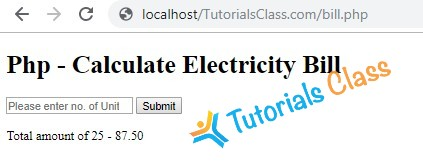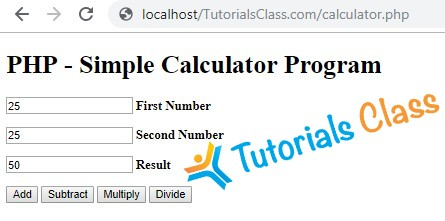# PHP Decision Making

PHP decision making statements allow us to make a decision based on some conditions.

PHP decision making is frequently used to perform tasks based on some calculations. Therefore, it is good to practice on these and understand how PHP If-Else, and switch statements works.

Here, you will find Assignments & Exercises related to PHP Variables. These exercises list contains exercise description and instructions. You need to write a program as per instructions. Then you can match it with our solution.

All PHP Decision Making and conditional statement based exercises are available in the form of PHP program description, instruction and their solution. These PHP programs can also be given as an assignment for PHP students.

## Write a program to calculate Electricity bill in PHP

Description:

You need to write a PHP program to calculate electricity bill using if-else conditions.

Conditions:

• For first 50 units – Rs. 3.50/unit
• For next 100 units – Rs. 4.00/unit
• For next 100 units – Rs. 5.20/unit
• For units above 250 – Rs. 6.50/unit
• You can use conditional statements.

View Solution/Program

``````<!DOCTYPE html>

<title>PHP - Calculate Electricity Bill</title>

<?php
\$result_str = \$result = '';
if (isset(\$_POST['unit-submit'])) {
\$units = \$_POST['units'];
if (!empty(\$units)) {
\$result = calculate_bill(\$units);
\$result_str = 'Total amount of ' . \$units . ' - ' . \$result;
}
}
/**
* To calculate electricity bill as per unit cost
*/
function calculate_bill(\$units) {
\$unit_cost_first = 3.50;
\$unit_cost_second = 4.00;
\$unit_cost_third = 5.20;
\$unit_cost_fourth = 6.50;

if(\$units <= 50) {
\$bill = \$units * \$unit_cost_first;
}
else if(\$units > 50 && \$units <= 100) {
\$temp = 50 * \$unit_cost_first;
\$remaining_units = \$units - 50;
\$bill = \$temp + (\$remaining_units * \$unit_cost_second);
}
else if(\$units > 100 && \$units <= 200) {
\$temp = (50 * 3.5) + (100 * \$unit_cost_second);
\$remaining_units = \$units - 150;
\$bill = \$temp + (\$remaining_units * \$unit_cost_third);
}
else {
\$temp = (50 * 3.5) + (100 * \$unit_cost_second) + (100 * \$unit_cost_third);
\$remaining_units = \$units - 250;
\$bill = \$temp + (\$remaining_units * \$unit_cost_fourth);
}
return number_format((float)\$bill, 2, '.', '');
}

?>

<body>
<div id="page-wrap">
<h1>Php - Calculate Electricity Bill</h1>

<form action="" method="post" id="quiz-form">
<input type="number" name="units" id="units" placeholder="Please enter no. of Units" />
<input type="submit" name="unit-submit" id="unit-submit" value="Submit" />
</form>

<div>
<?php echo '<br />' . \$result_str; ?>
</div>
</div>
</body>
</html>``````
Tutorials Class - Output Window## Write a simple calculator program in PHP using switch case

Description:

You need to write a simple calculator program in PHP using switch case.

Operations:

• Subtraction
• Multiplication
• Division

View Solution/Program

``````<!DOCTYPE html>

<title>Simple Calculator Program in PHP - Tutorials Class</title>

<?php
\$first_num = \$_POST['first_num'];
\$second_num = \$_POST['second_num'];
\$operator = \$_POST['operator'];
\$result = '';
if (is_numeric(\$first_num) && is_numeric(\$second_num)) {
switch (\$operator) {
\$result = \$first_num + \$second_num;
break;
case "Subtract":
\$result = \$first_num - \$second_num;
break;
case "Multiply":
\$result = \$first_num * \$second_num;
break;
case "Divide":
\$result = \$first_num / \$second_num;
}
}

?>

<body>
<div id="page-wrap">
<h1>PHP - Simple Calculator Program</h1>
<form action="" method="post" id="quiz-form">
<p>
<input type="number" name="first_num" id="first_num" required="required" value="<?php echo \$first_num; ?>" /> <b>First Number</b>
</p>
<p>
<input type="number" name="second_num" id="second_num" required="required" value="<?php echo \$second_num; ?>" /> <b>Second Number</b>
</p>
<p>
</p>
<input type="submit" name="operator" value="Subtract" />
<input type="submit" name="operator" value="Multiply" />
<input type="submit" name="operator" value="Divide" />
</form>
</div>
</body>
</html>``````
Tutorials Class - Output Window## Write a PHP program to check if a person is eligible to vote

#### Description:

Write a PHP program to check if a person is eligible to vote or not.

##### Click to View Solution/Program.
``````<?php
function check_vote() //function has been declared
{
\$name = "Rakesh";
\$age = 19;
if (\$age >= 18) {
echo \$name . ", you Are Eligible For Vote";
} else {
echo \$name . ", you are not eligible for vote. ";
}
}
check_vote(); //function has been called

?>``````
Tutorials Class - Output Window

You Are Eligible For Vote

## Write a PHP program to check whether a number is positive, negative or zero

#### Description:

Write a PHP program to check whether a number is positive, negative or zero.

#### Instructions:

• You can use if else conditions.
• You should use appropriate PHP Operators.
• Also check if it not a numeric value.

View Solution/Program

``````<?php
\$number = 324; // enter any number of your choice here
if (\$number > 0) // condition for positive numbers
{
echo \$number . " is a positive number";
} else if (\$number < 0) // condition for negative number
{
echo \$number . " is a negative number ";
} else if (\$number == 0) // condition for zero
{
echo "You have entered zero";
} else {
echo " please enter a numeric value";
}
?>``````
Tutorials Class - Output Window

324 is a positive number